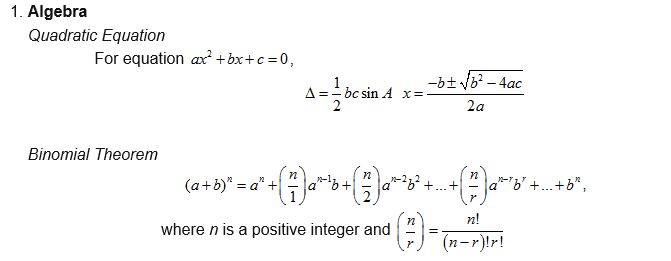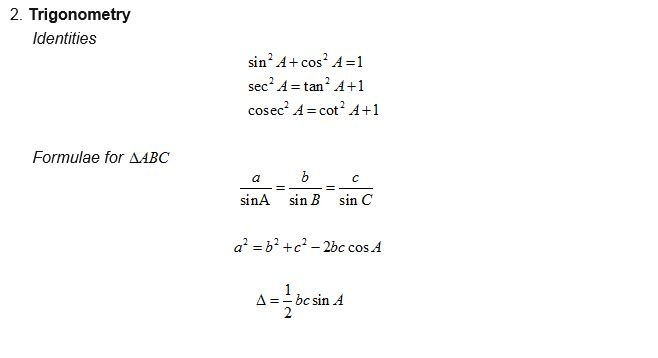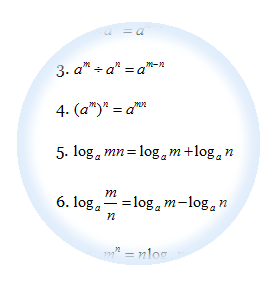All posts by Mr LowA full time tutor from Malaysia, passionate about teaching Mathematics for PMR Maths, SPM Additional Maths, SPM Modern Maths and International School Maths included SAT,GCSE, IGCSE syllabus.

Formulae that will be given in IGCSE 0606 Additional Mathematic

These are the formulae that will be given in the IGCSE 0606 Additional Maths Exam to help you to answer the Questions. Basically there are divided into 2 categories:Indices and Logarithms

Indices$\text{Positive Index: }{{a}^{n}},a\ne 0$$eg:{{2}^{3}},{{4}^{2}}$$\text{Negative Index: }{{a}^{-n}}=\frac{1}{{{a}^{n}}}$$eg:{{2}^{-3}}=\frac{1}{{{2}^{3}}}=\frac{1}{8}$$\text{Zero Index: }{{a}^{0}}=1,a\ne 0$$eg:{{2}^{0}}=1$

Fractional Indices:${{a}^{\frac{1}{n}}}=\sqrt[n]{a},a\ne 0$$eg:{{8}^{\frac{1}{3}}}=\sqrt{8}=2$${{a}^{\frac{m}{n}}}={{(\sqrt[n]{a})}^{m}},a\ne 0$$eg:{{8}^{\frac{2}{3}}}={{(\sqrt{8})}^{2}}={{(2)}^{2}}=4$

Law of Indices:$\text{Rule 1: }{{a}^{m}}\times {{a}^{n}}={{a}^{m+n}}$$eg:{{2}^{2}}\times {{2}^{3}}={{2}^{2+3}}={{2}^{5}}=32$$\text{Rule 2: }{{a}^{m}}\div {{a}^{n}}={{a}^{m-n}}$$eg:{{2}^{4}}\div {{2}^{2}}={{2}^{4-2}}={{2}^{2}}=4$$\text{Rule 3: }{{({{a}^{m}})}^{n}}={{a}^{m\times n}}$$eg:{{({{2}^{2}})}^{3}}={{2}^{2\times 3}}={{2}^{6}}=64$

Formulae that will be given in SPM Add MathsThese are the formulae that will be given to help you to answer the SPM Additional Maths Questions. However, more important is that you know how to apply those formulaes to solve your questions. Buy some exercises from the bookstore and work on the questions everyday and you will master all those formulaes. Basically there are divided into 5 categories:

1. Algebra
2. Calculus
3. Statistics
4. Geometry
5. Trigonometry

SPM Questions for Simultaneous Equations

At least one simultaneous equations question (5 marks) will be coming out in the SPM paper 2, section A.

1. Substitution (SPM 2005, SPM2007)
2. Using Formulae (SPM 2006, SPM 2009)

1. The Basic of quadratic functions (SPM 2010)
2. Determine max and min values of quadratic function (SPM2009)
3. How to sketch the graph of quadratic functions (SPM2010)
4. How to find the range of values of x in Quadratic inequalities (2007, 2006)

We will discuss some pass year SPM exam questions based on:

• Solve the quadratic equations – SPM 2003, SPM 2005
• Form a quadratic equation – SPM 2009
• Determine the conditions for the type of roots – SPM2010

3 Basic Techniques in Solving Quadratic Equation Questions

In this chapter we will learn 3 most basic techniques on how to:

3. Determine the conditions for the type of roots.

SPM Questions for functions

Frequently Asked Questions in SPM for Functions

We will discuss some pass year SPM exam questions based on the four concepts :

Relations – SPM 2010
Functions – SPM 2007
Composite Functions – SPM 2007
Composite & Inverse Functions – SPM 2010